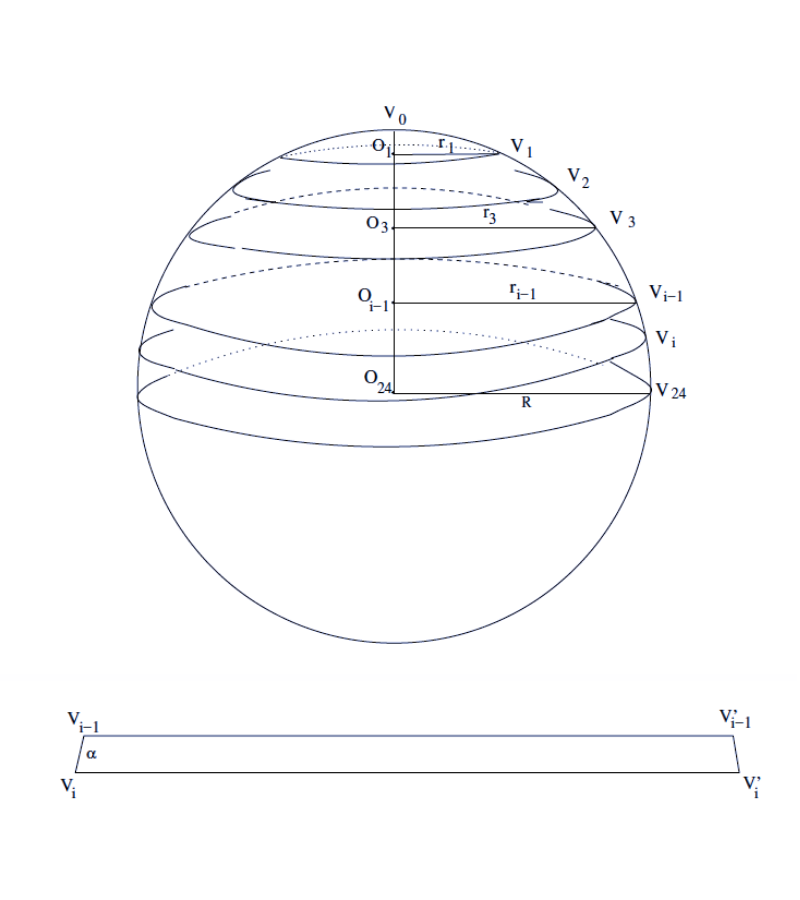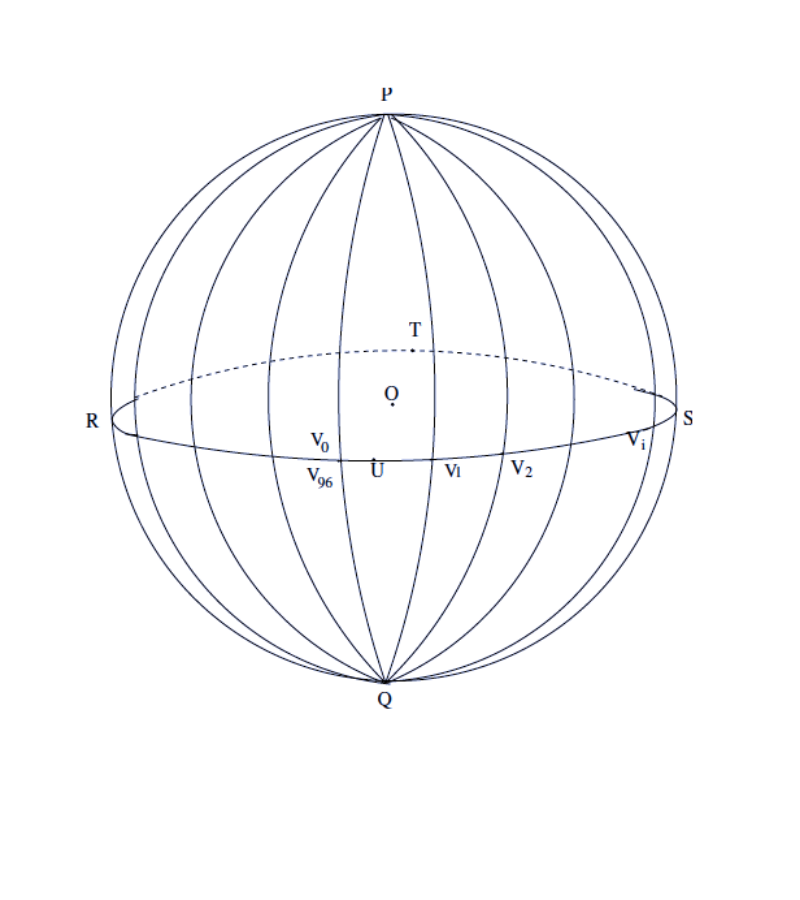Methods of Archimedes and Bhāskara to Help Teaching

Researchers compare how the Greek and Indian mathematicians measured the surface area of a sphere

Mathematics at school was rote learning for a majority of us, and the formula for the surface area of a sphere was one out of the several we learnt by heart. We would keep employing it in a variety of contexts with no understanding of how we arrived at that magic formula. But teachers can use methods from ancient mathematical studies to help students visualise the surface of the sphere in different ways and understand the area calculation in an intuitive way. In a study published in the Journal of the Indian Mathematical Society, researchers at the Indian Institute of Technology Bombay, led by Prof K Ramasubramanian, compared the very first methods that Greek and Indian mathematicians of the old era used to find the surface area of a sphere. The researchers bring up some interesting aspects regarding how those approaches could help to teach the topic.

In modern mathematics, the surface area of a sphere is calculated using integral calculus, but its formula was known several centuries before Newton and Leibniz developed calculus in the 17th century. Archimedes is thought to be the first person to have worked out the surface area of a sphere in the 3rd century BCE, in his work On the Sphere and Cylinder. Interestingly, much like the ancient Greeks, mathematicians in India too attempted to find areas and volumes of geometrical figures. Some of the earliest references to finding areas and attempting area-preserving transformations can be found in Sulva-sutra texts (c.800 BCE), which primarily deal with the construction of altars.

Indian mathematicians tackled the problems related to the circle and sphere at least since the 5th century CE when Āryabhaṭa I gave the correct formula for the area of a circle in the Āryabhaṭīya. But the first Indian text to address the problem of finding the surface area of a sphere is believed to be the Pāṭīgaṇita of Lalla, written in the 8th century CE. Although it has been lost, Bhāskara has quoted and critiqued Lalla’s results and found them erroneous. In the mid-10th century CE, Āryabhaṭa II gave an interesting explanation to calculate the surface area of a sphere in the Mahā-siddhānta when he said that one can imagine a net spread over a ball to find the surface area of the earth. He stated that the area is the circumference multiplied by the diameter, but from the literature that survives, we have no clue how he demonstrated the result.

Using the same idea of the net spread over a ball, Bhāskara provided the correct formula for the surface area of a sphere in his most famous work, Līlāvatī, in the 12th century CE. He followed it up by providing two different methods for carrying out the calculation, in the Siddhāntaśiromaṇi, and its auto-commentary the Vāsanābhāṣya. In addition to being important from a mathematical standpoint, the methods present helpful approaches from the perspective of teaching the topic.

In the first method, described in the Vāsanābhāṣya, Bhāskara suggested dividing the sphere into a series of strips, like how one would slice a tomato. The pieces nearer to the centre are larger compared to those closer to the poles. Each strip, when peeled and unrolled, forms a trapezium, whose area can be calculated using a known formula. The breadth of each piece depends on the number of slices, and the length depends on how close it was to the centre. Using an established relationship between the distance of the strip from the centre and its dimensions, its area can be calculated. The sum of the areas of all strips gives us the surface area of the sphere. This approach is similar to cutting the surface of the Earth along the latitudes.

The second method, described in the Siddhāntaśiromaṇi and expanded upon in the Vāsanābhāṣyais similar in principle. But, instead of slicing the sphere like a tomato, imagine cutting it like an apple, or to go by Bhāskara’s example, similar to a gooseberry (āmalaka) or āmla. The slices can be peeled to form crescent-like shapes of the same size. Bhāskara further cut the crescents along latitude lines to obtain triangles at the ends and trapezia in the middle and used known formulae to calculate their area. Summing the areas of all the triangles and trapezia gives the surface area of the sphere. This method is like cutting the surface of the Earth along the longitudes.

On the Greek side, Archimedes stated that the sphere is four times that of a cone which has the great circle of the sphere as a base, and the radius of the sphere as the height. He presented a rigorous proof for the formula he suggested, employing a technique known as reductio ad absurdum. “Archimedes meticulously structures his arguments by first laying out a number of axioms and assumptions, and then slowly builds towards the surface area of a sphere over thirty-three propositions, proving each in turn before moving on to the next”, say the authors.

First, he showed that a solid body with any number of sides that can be contained within the sphere has a surface area less than 4 times the area of the largest circle that can be drawn on a sphere. Therefore, the area of the sphere cannot be less than 4 times the area of the largest circle that can be drawn on it. He then showed that a solid body with any number of sides that can contain the sphere within it has a surface area greater than 4 times the area of the largest circle that can be drawn on a sphere. Hence, the area of the sphere cannot be greater than 4 times the area of the largest circle that can be drawn on it. It follows that a sphere must have an area exactly equal to 4 times the largest circle that can be drawn on it.

Highlighting the differences between the two approaches, the researchers suggest that those are driven by the underlying motivation; Bhāskara’s methods provide a more practical and demonstrative proof, Archimedes presents a more theoretical explanation. Bhāskara’s methods involve dividing the surface of the sphere into known geometrical shapes and adding their areas, an approach that is much easier for young minds to understand and visualise in addition to being useful for solving practical problems. Bhāskara uses simple multiplicative and additive expressions, easier to understand for students familiar with formulae for an area being some variation of base times height.

The researchers feel that among the two approaches, Bhāskara’s methods seem better suited to introduce the subject matter to students. Since many of Bhāskara’s works have similar lucidity of thought, they suggest adding them in the prescribed mathematics syllabus in schools. The methods of Archimedes, on the other hand, will appeal to the students of advanced mathematics due to the meticulous approach of starting with axioms and slowly building up arguments proposition by proposition. “His (Archimedes) approach highlights some important lessons in how to approach a challenging mathematical problem”, opine the authors.

“The Indian mathematicians of yore combined vivid imagination and poetic charm to teach mathematical concepts and relations to students. They rooted their examples in popular culture and placed a great emphasis on practical demonstrations of their proofs to capture the interest of young minds. Re-adopting such techniques and rooting education in a culture and language familiar to young students would generate more interest in mathematics and improve educational outcomes”, says Dr Aditya Kolachana, one of the authors of the study. As a next step, the authors advocate further research into Bhāskara’s works, which they believe are not yet “sufficiently studied compared to those of Archimedes”.

 Author(s) of the research paper K. Mahesh, Aditya Kolachana, K. Ramasubramanian, Indian Institute of Technology Bombay, Powai, Mumbai. Article was written by Vimal Simha and Arati Halbe Graphics/Image by Annie Santamaria Image usage rights Gubbi Page link
Research Domain: